# Hierarchied Categories Abelian categories hierarchied abelian categories graded

• Slides: 45Hierarchied Categories Abelian categories, hierarchied abelian categories, graded hierarchied tensor categories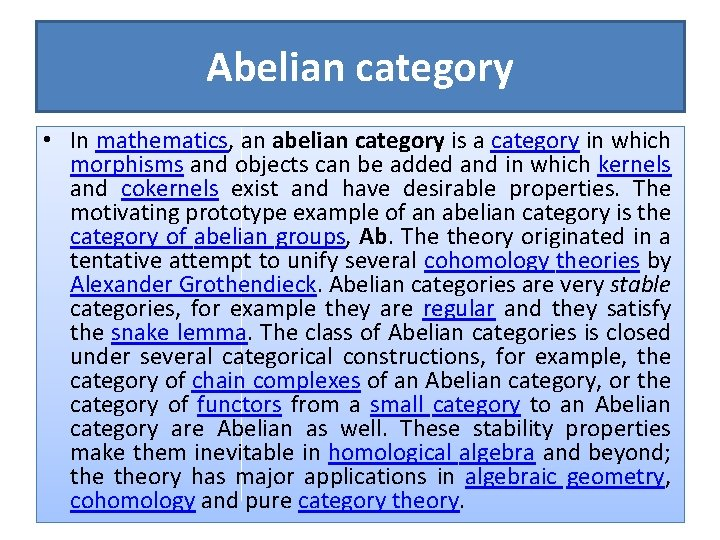Abelian category • In mathematics, an abelian category is a category in which morphisms and objects can be added and in which kernels and cokernels exist and have desirable properties. The motivating prototype example of an abelian category is the category of abelian groups, Ab. The theory originated in a tentative attempt to unify several cohomology theories by Alexander Grothendieck. Abelian categories are very stable categories, for example they are regular and they satisfy the snake lemma. The class of Abelian categories is closed under several categorical constructions, for example, the category of chain complexes of an Abelian category, or the category of functors from a small category to an Abelian category are Abelian as well. These stability properties make them inevitable in homological algebra and beyond; theory has major applications in algebraic geometry, cohomology and pure category theory.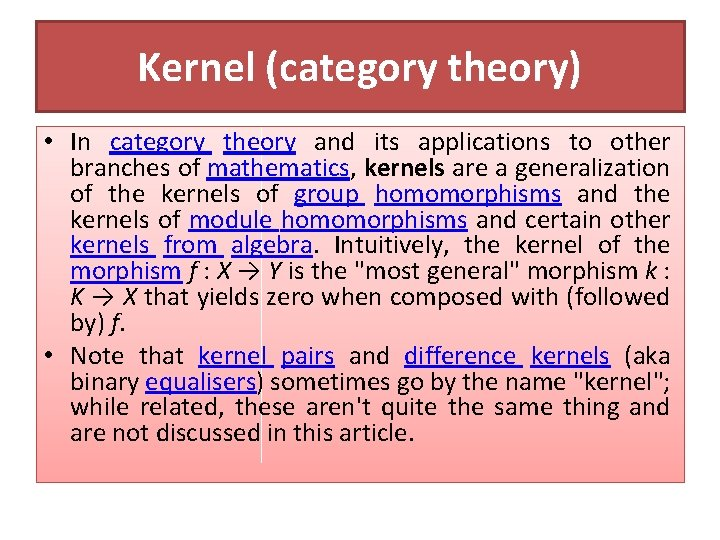Kernel (category theory) • In category theory and its applications to other branches of mathematics, kernels are a generalization of the kernels of group homomorphisms and the kernels of module homomorphisms and certain other kernels from algebra. Intuitively, the kernel of the morphism f : X → Y is the "most general" morphism k : K → X that yields zero when composed with (followed by) f. • Note that kernel pairs and difference kernels (aka binary equalisers) sometimes go by the name "kernel"; while related, these aren't quite the same thing and are not discussed in this article.Definition of kernel • • Let C be a category. In order to define a kernel in the general categorytheoretical sense, C needs to have zero morphisms. In that case, if f : X → Y is an arbitrary morphism in C, then a kernel of f is an equaliser of f and the zero morphism from X to Y. In symbols: ker(f) = eq(f, 0 XY) To be more explicit, the following universal property can be used. A kernel of f is any morphism k : K → X such that: f o k is the zero morphism from K to Y; Given any morphism k′ : K′ → X such that f o k′ is the zero morphism, there is a unique morphism u : K′ → K such that k o u = k'.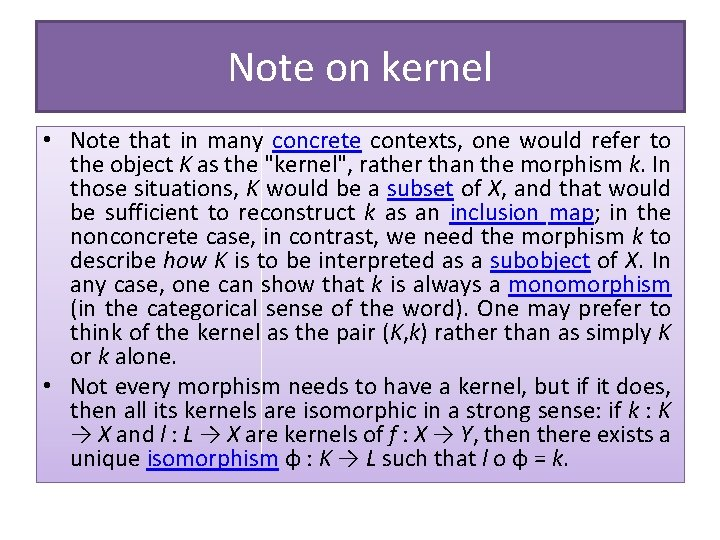Note on kernel • Note that in many concrete contexts, one would refer to the object K as the "kernel", rather than the morphism k. In those situations, K would be a subset of X, and that would be sufficient to reconstruct k as an inclusion map; in the nonconcrete case, in contrast, we need the morphism k to describe how K is to be interpreted as a subobject of X. In any case, one can show that k is always a monomorphism (in the categorical sense of the word). One may prefer to think of the kernel as the pair (K, k) rather than as simply K or k alone. • Not every morphism needs to have a kernel, but if it does, then all its kernels are isomorphic in a strong sense: if k : K → X and l : L → X are kernels of f : X → Y, then there exists a unique isomorphism φ : K → L such that l o φ = k.Examples of kernel • Kernels are familiar in many categories from abstract algebra, such as the category of groups or the category of (left) modules over a fixed ring (including vector spaces over a fixed field). To be explicit, if f : X → Y is a homomorphism in one of these categories, and K is its kernel in the usual algebraic sense, then K is a subalgebra of X and the inclusion homomorphism from K to X is a kernel in the categorical sense. • Note that in the category of monoids, category-theoretic kernels exist just as for groups, but these kernels don't carry sufficient information for algebraic purposes. Therefore, the notion of kernel studied in monoid theory is slightly different. Conversely, in the category of rings, there are no kernels in the category-theoretic sense; indeed, this category does not even have zero morphisms. Nevertheless, there is still a notion of kernel studied in ring theory. See Relationship to algebraic kernels below for the resolution of this paradox. • In the category of pointed topological spaces, if f : X → Y is a continuous pointed map, then the preimage of the distinguished point, K, is a subspace of X. The inclusion map of K into X is the categorical kernel of f. • We have plenty of algebraic examples; now we should give examples of kernels in categories from topology and functional analysis.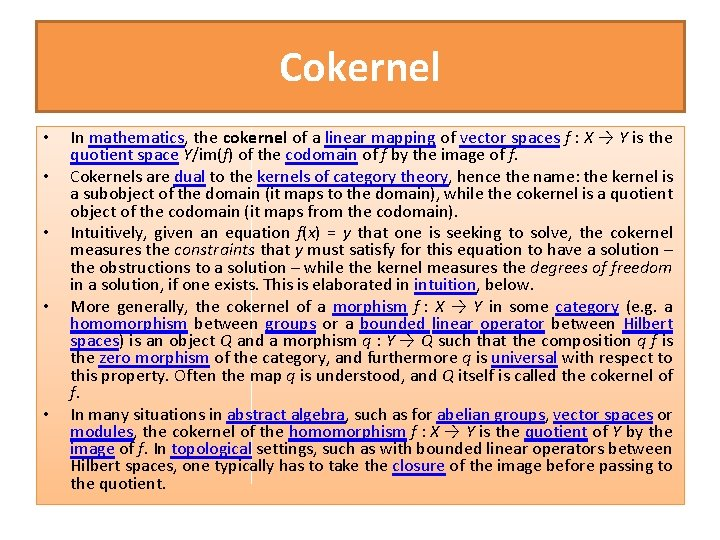Cokernel • • • In mathematics, the cokernel of a linear mapping of vector spaces f : X → Y is the quotient space Y/im(f) of the codomain of f by the image of f. Cokernels are dual to the kernels of category theory, hence the name: the kernel is a subobject of the domain (it maps to the domain), while the cokernel is a quotient object of the codomain (it maps from the codomain). Intuitively, given an equation f(x) = y that one is seeking to solve, the cokernel measures the constraints that y must satisfy for this equation to have a solution – the obstructions to a solution – while the kernel measures the degrees of freedom in a solution, if one exists. This is elaborated in intuition, below. More generally, the cokernel of a morphism f : X → Y in some category (e. g. a homomorphism between groups or a bounded linear operator between Hilbert spaces) is an object Q and a morphism q : Y → Q such that the composition q f is the zero morphism of the category, and furthermore q is universal with respect to this property. Often the map q is understood, and Q itself is called the cokernel of f. In many situations in abstract algebra, such as for abelian groups, vector spaces or modules, the cokernel of the homomorphism f : X → Y is the quotient of Y by the image of f. In topological settings, such as with bounded linear operators between Hilbert spaces, one typically has to take the closure of the image before passing to the quotient.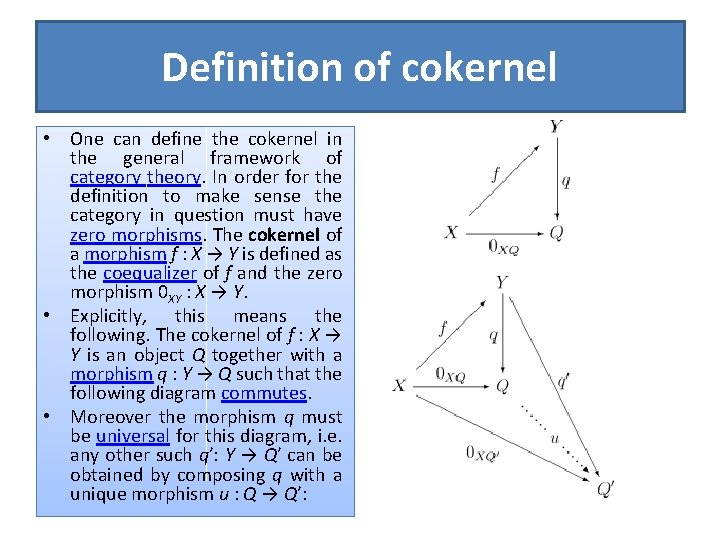Definition of cokernel • One can define the cokernel in the general framework of category theory. In order for the definition to make sense the category in question must have zero morphisms. The cokernel of a morphism f : X → Y is defined as the coequalizer of f and the zero morphism 0 XY : X → Y. • Explicitly, this means the following. The cokernel of f : X → Y is an object Q together with a morphism q : Y → Q such that the following diagram commutes. • Moreover the morphism q must be universal for this diagram, i. e. any other such q′: Y → Q′ can be obtained by composing q with a unique morphism u : Q → Q′: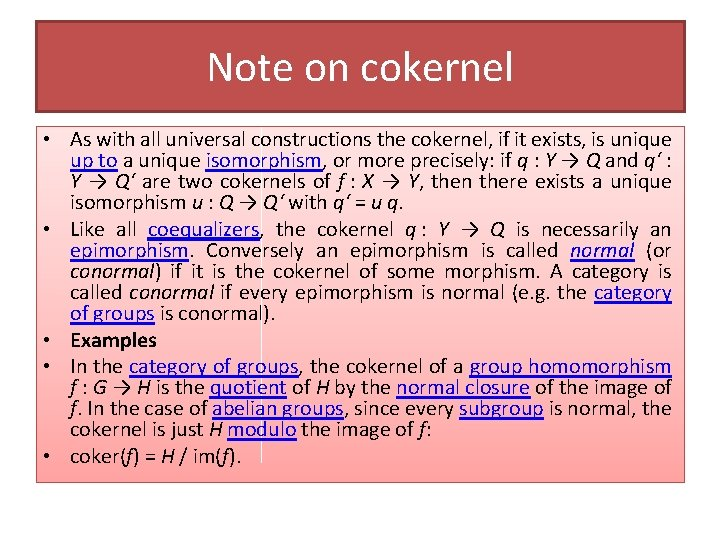Note on cokernel • As with all universal constructions the cokernel, if it exists, is unique up to a unique isomorphism, or more precisely: if q : Y → Q and q‘ : Y → Q‘ are two cokernels of f : X → Y, then there exists a unique isomorphism u : Q → Q‘ with q‘ = u q. • Like all coequalizers, the cokernel q : Y → Q is necessarily an epimorphism. Conversely an epimorphism is called normal (or conormal) if it is the cokernel of some morphism. A category is called conormal if every epimorphism is normal (e. g. the category of groups is conormal). • Examples • In the category of groups, the cokernel of a group homomorphism f : G → H is the quotient of H by the normal closure of the image of f. In the case of abelian groups, since every subgroup is normal, the cokernel is just H modulo the image of f: • coker(f) = H / im(f).Special cases of cokernel • In a preadditive category, it makes sense to add and subtract morphisms. In such a category, the coequalizer of two morphisms f and g (if it exists) is just the cokernel of their difference: • coeq(f, g) = coker(g - f). In a pre-abelian category (a special kind of preadditive category) the existence of kernels and cokernels is guaranteed. In such categories the image and coimage of a morphism f are given by • im(f) = ker(coker f) coim(f) = coker(ker f). Abelian categories are even better behaved with respect to cokernels. In particular, every abelian category is conormal (and normal as well). That is, every epimorphism e : A → B can be written as the cokernel of some morphism. Specifically, e is the cokernel of its own kernel: • e = coker(ker e).Intuition • • The cokernel can be thought of as the space of constraints that an equation must satisfy, as the space of obstructions, just as the kernel is the space of solutions. Formally, one may connect the kernel and the cokernel by the exact sequence These can be interpreted thus: given a linear equation T(v) = w to solve, the kernel is the space of solutions to the homogeneous equation T(v) = 0, and its dimension is the number of degrees of freedom in a solution, if it exists; the cokernel is the space of constraints that must be satisfied if the equation is to have a solution, and its dimension is the number of constraints that must be satisfied for the equation to have a solution. The dimension of the cokernel plus the dimension of the image (the rank) add up to the dimension of the target space, as the dimension of the quotient space W / T(V) is simply the dimension of the space minus the dimension of the image. As a simple example, consider the map given by T(x, y) = (0, y). Then for an equation T(x, y) = (a, b) to have a solution, we must have a = 0 (one constraint), and in that case the solution space is (x, b), or equivalently stated, (0, b) + (x, 0), (one degree of freedom). The kernel may be expressed as the subspace (x, 0) < V: the value of x is the freedom in a solution – while the cokernel may be expressed via the map given a vector (a, b), the value of a is the obstruction to there being a solution.Initial and terminal objects • "Terminal element" redirects here. For the project management concept, see work breakdown structure. • In category theory, an abstract branch of mathematics, an initial object of a category C is an object I in C such that for every object X in C, there exists precisely one morphism I → X. The dual notion is that of a terminal object (also called terminal element): T is terminal if for every object X in C there exists a single morphism X → T. Initial objects are also called coterminal or universal, and terminal objects are also called final. • If an object is both initial and terminal, it is called a zero object or null object.Examples of initial and terminal objs • • • The empty set is the unique initial object in the category of sets; every oneelement set (singleton) is a terminal object in this category; there are no zero objects. Similarly, the empty space is the unique initial object in the category of topological spaces; every one-point space is a terminal object in this category. In the category of non-empty sets, there are no initial objects. The singletons are not initial: while every non-empty set admits a function from a singleton, this function is in general not unique. In the category of groups, any trivial group is a zero object. The same is true for the categories of abelian groups, modules over a ring, and vector spaces over a field. This is the origin of the term "zero object". In the category of semigroups, the empty semigroup is an initial object and any singleton semigroup is a terminal object. There are no zero objects. In the subcategory of monoids, however, every trivial monoid (consisting of only the identity element) is a zero object. In the category of pointed sets (whose objects are non-empty sets together with a distinguished element; a morphism from (A, a) to (B, b) being a function f : A → B with f(a) = b), every singleton is a zero object. Similarly, in the category of pointed topological spaces, every singleton is a zero object.More examples • • • In the category of rings with unity and unity-perserving morphisms, the ring of integers Z is an initial object. The trivial ring consisting only of a single element 0=1 is a terminal object. In the category of general rings with homomorphisms, the trivial ring is a zero object. In the category of fields, there are no initial or terminal objects. However, in the subcategory of fields of characteristic p, the prime field of characteristic p forms an initial object. Any partially ordered set (P, ≤) can be interpreted as a category: the objects are the elements of P, and there is a single morphism from x to y if and only if x ≤ y. This category has an initial object if and only if P has a least element; it has a terminal object if and only if P has a greatest element. If a monoid is considered as a category with a single object, this object is neither initial or terminal unless the monoid is trivial, in which case it is both. In the category of graphs, the null graph (without vertices and edges) is an initial object. The graph with a single vertex and a single loop is terminal. The category of simple graphs does not have a terminal object. Similarly, the category of all small categories with functors as morphisms has the empty category as initial object and the category 1 (with a single object and morphism) as terminal object.• • • Any topological space X can be viewed as a category by taking the open sets as objects, and a single morphism between two open sets U and V if and only if U ⊂ V. The empty set is the initial object of this category, and X is the terminal object. This is a special case of the case "partially ordered set", mentioned above. Take P: = the set of open subsets If X is a topological space (viewed as a category as above) and C is some small category, we can form the category of all contravariant functors from X to C, using natural transformations as morphisms. This category is called the category of presheaves on X with values in C. If C has an initial object c, then the constant functor which sends every open set to c is an initial object in the category of presheaves. Similarly, if C has a terminal object, then the corresponding constant functor serves as a terminal presheaf. In the category of schemes, Spec(Z) the prime spectrum of the ring of integers is a terminal object. The empty scheme (equal to the prime spectrum of the trivial ring) is an initial object. If we fix a homomorphism f : A → B of abelian groups, we can consider the category C consisting of all pairs (X, φ) where X is an abelian group and φ : X → A is a group homomorphism with f φ = 0. A morphism from the pair (X, φ) to the pair (Y, ψ) is defined to be a group homomorphism r : X → Y with the property ψ r = φ. The kernel of f is a terminal object in this category; this is nothing but a reformulation of the universal property of kernels. With an analogous construction, the cokernel of f can be seen as an initial object of a suitable category. In the category of interpretations of an algebraic model, the initial object is the initial algebra, the interpretation that provides as many distinct objects as the model allows and no more.Properties of initials and terminals • Existence and uniqueness • Initial and terminal objects are not required to exist in a given category. However, if they do exist, they are essentially unique. Specifically, if I 1 and I 2 are two different initial objects, then there is a unique isomorphism between them. Moreover, if I is an initial object then any object isomorphic to I is also an initial object. The same is true for terminal objects. • For complete categories there is an existence theorem for initial objects. Specifically, a (locally small) complete category C has an initial object if and only if there exist a set I (not a proper class) and an I-indexed family (Ki) of objects of C such that for any object X of C there at least one morphism Ki → X for some i ∈ I. • Other properties • The endomorphism monoid of an initial or terminal object I is trivial: End(I) = Hom(I, I) = { id. I }. • If a category C has a zero object 0 then for any pair of objects X and Y in C the unique composition X → 0 → Y is a zero morphism from X to Y.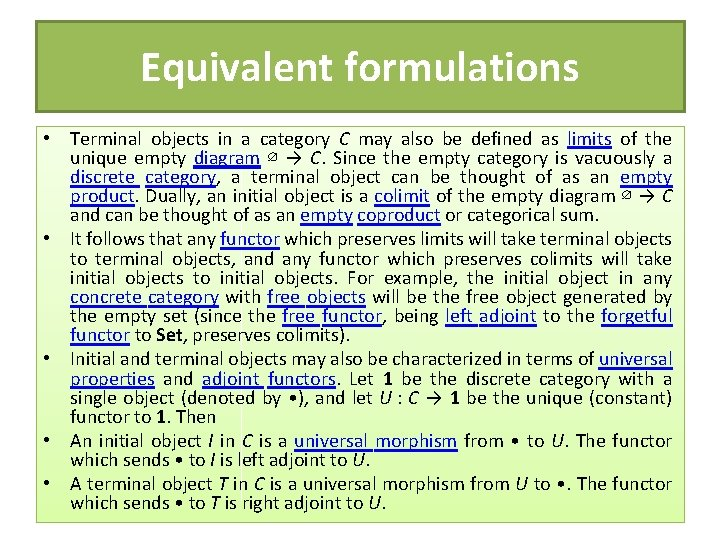Equivalent formulations • Terminal objects in a category C may also be defined as limits of the unique empty diagram ∅ → C. Since the empty category is vacuously a discrete category, a terminal object can be thought of as an empty product. Dually, an initial object is a colimit of the empty diagram ∅ → C and can be thought of as an empty coproduct or categorical sum. • It follows that any functor which preserves limits will take terminal objects to terminal objects, and any functor which preserves colimits will take initial objects to initial objects. For example, the initial object in any concrete category with free objects will be the free object generated by the empty set (since the free functor, being left adjoint to the forgetful functor to Set, preserves colimits). • Initial and terminal objects may also be characterized in terms of universal properties and adjoint functors. Let 1 be the discrete category with a single object (denoted by • ), and let U : C → 1 be the unique (constant) functor to 1. Then • An initial object I in C is a universal morphism from • to U. The functor which sends • to I is left adjoint to U. • A terminal object T in C is a universal morphism from U to • . The functor which sends • to T is right adjoint to U.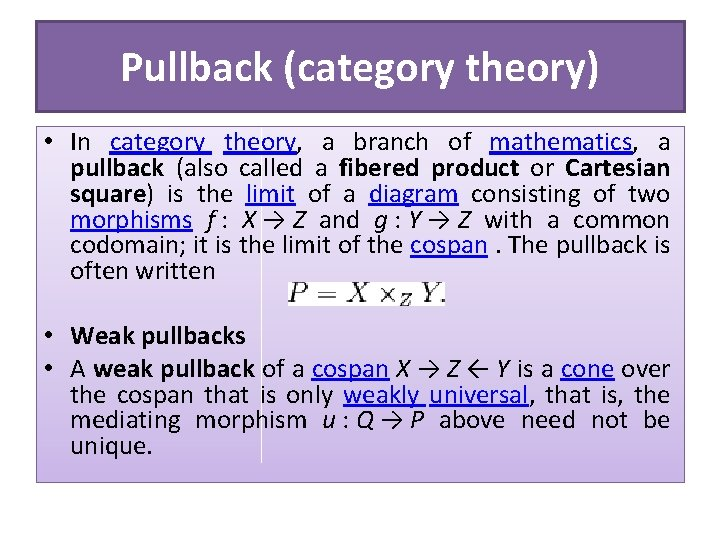Pullback (category theory) • In category theory, a branch of mathematics, a pullback (also called a fibered product or Cartesian square) is the limit of a diagram consisting of two morphisms f : X → Z and g : Y → Z with a common codomain; it is the limit of the cospan. The pullback is often written • Weak pullbacks • A weak pullback of a cospan X → Z ← Y is a cone over the cospan that is only weakly universal, that is, the mediating morphism u : Q → P above need not be unique.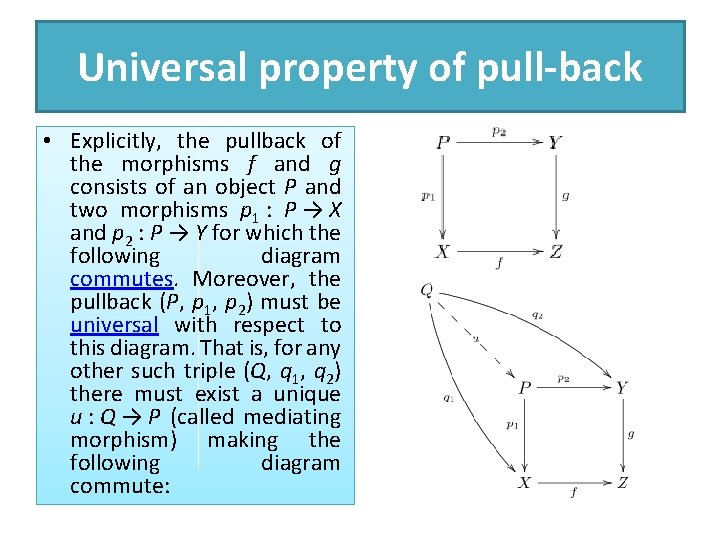Universal property of pull-back • Explicitly, the pullback of the morphisms f and g consists of an object P and two morphisms p 1 : P → X and p 2 : P → Y for which the following diagram commutes. Moreover, the pullback (P, p 1, p 2) must be universal with respect to this diagram. That is, for any other such triple (Q, q 1, q 2) there must exist a unique u : Q → P (called mediating morphism) making the following diagram commute:Examples of pull-back • In the category of sets, a pullback of f and g is given by the set • • together with the restrictions of the projection maps π1 and π2 to X × Z Y. Alternatively one may view the pullback in Set asymmetrically: • where is the disjoint (tagged) union of sets (the involved sets are not disjoint on their own unless f resp. g is injective). In the first case, the projection π1 extracts the x index while π2 forgets the index, leaving elements of Y. This example motivates another way of characterizing the pullback: as the equalizer of the morphisms f o p 1, g o p 2 : X × Y → Z where X × Y is the binary product of X and Y and p 1 and p 2 are the natural projections. This shows that pullbacks exist in any category with binary products and equalizers. In fact, by the existence theorem for limits, all finite limits exist in a category with a terminal object, binary products and equalizers. Another example of a pullback comes from theory of fiber bundles: given a bundle map π : E → B and a continuous map f : X → B, the pullback X ×B E is a fiber bundle over X called the pullback bundle. The associated commutative diagram is a morphism of fiber bundles. In any category with a terminal object Z, the pullback X ×Z Y is just the ordinary product X × Y. • • •Preimages • • • Preimages of sets under functions can be described as pullbacks as follows: Suppose f : A → B and B 0 ⊆ B. Let g be the inclusion map B 0 ↪ B. Then a pullback of f and g (in Set) is given by the preimage f-1 [ B 0 ] together with the inclusion of the preimage in A f-1 [ B 0 ] ↪ A and the restriction of f to f-1 [ B 0 ] → B 0. Properties Whenever X ×ZY exists, then so does Y ×Z X and there is an isomorphism X ×Z Y Y ×ZX. Monomorphisms are stable under pullback: if the arrow f above is monic, then so is the arrow p 2. For example, in the category of sets, if X is a subset of Z, then, for any g : Y → Z, the pullback X ×Z Y is the inverse image of X under g. Isomorphisms are also stable, and hence, for example, X ×X Y Y for any map Y → X. Any category with fibre products (pull backs) and products has equalizers.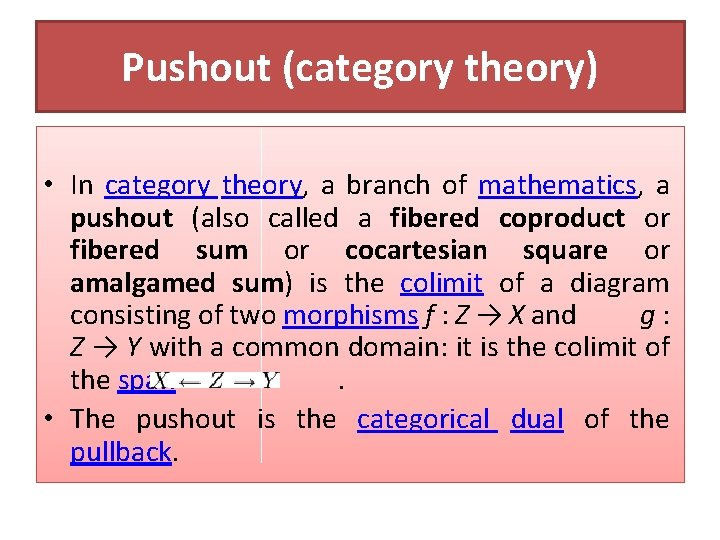Pushout (category theory) • In category theory, a branch of mathematics, a pushout (also called a fibered coproduct or fibered sum or cocartesian square or amalgamed sum) is the colimit of a diagram consisting of two morphisms f : Z → X and g : Z → Y with a common domain: it is the colimit of the span . • The pushout is the categorical dual of the pullback.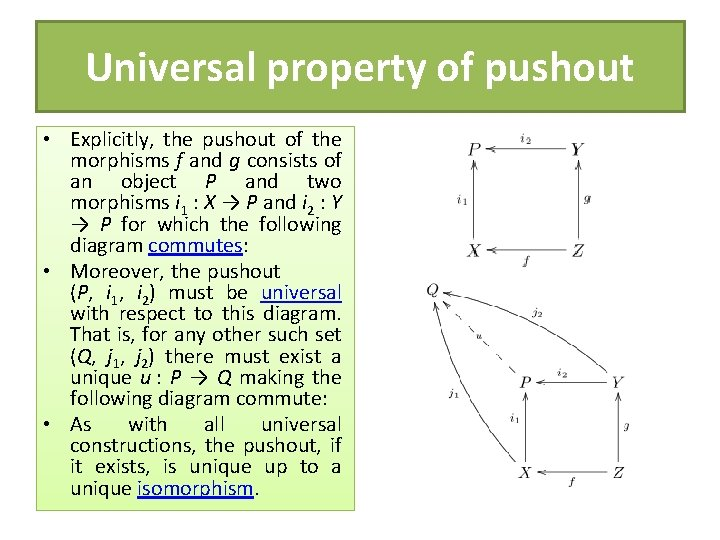Universal property of pushout • Explicitly, the pushout of the morphisms f and g consists of an object P and two morphisms i 1 : X → P and i 2 : Y → P for which the following diagram commutes: • Moreover, the pushout (P, i 1, i 2) must be universal with respect to this diagram. That is, for any other such set (Q, j 1, j 2) there must exist a unique u : P → Q making the following diagram commute: • As with all universal constructions, the pushout, if it exists, is unique up to a unique isomorphism.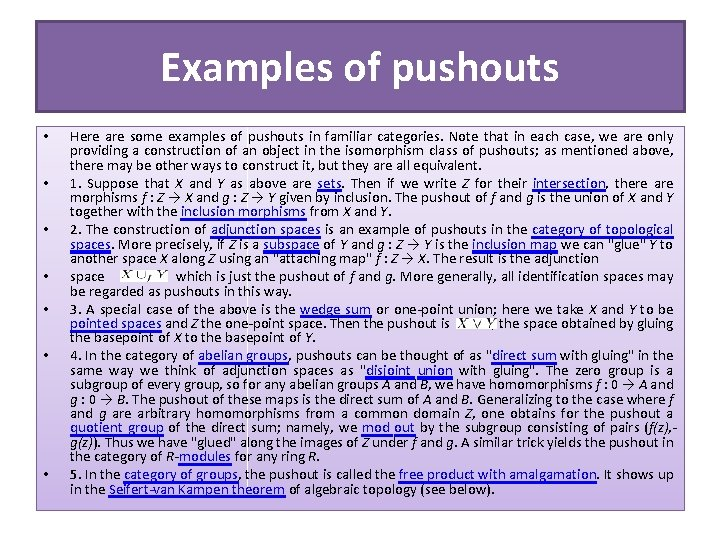Examples of pushouts • • Here are some examples of pushouts in familiar categories. Note that in each case, we are only providing a construction of an object in the isomorphism class of pushouts; as mentioned above, there may be other ways to construct it, but they are all equivalent. 1. Suppose that X and Y as above are sets. Then if we write Z for their intersection, there are morphisms f : Z → X and g : Z → Y given by inclusion. The pushout of f and g is the union of X and Y together with the inclusion morphisms from X and Y. 2. The construction of adjunction spaces is an example of pushouts in the category of topological spaces. More precisely, if Z is a subspace of Y and g : Z → Y is the inclusion map we can "glue" Y to another space X along Z using an "attaching map" f : Z → X. The result is the adjunction space which is just the pushout of f and g. More generally, all identification spaces may be regarded as pushouts in this way. 3. A special case of the above is the wedge sum or one-point union; here we take X and Y to be pointed spaces and Z the one-point space. Then the pushout is , the space obtained by gluing the basepoint of X to the basepoint of Y. 4. In the category of abelian groups, pushouts can be thought of as "direct sum with gluing" in the same way we think of adjunction spaces as "disjoint union with gluing". The zero group is a subgroup of every group, so for any abelian groups A and B, we have homomorphisms f : 0 → A and g : 0 → B. The pushout of these maps is the direct sum of A and B. Generalizing to the case where f and g are arbitrary homomorphisms from a common domain Z, one obtains for the pushout a quotient group of the direct sum; namely, we mod out by the subgroup consisting of pairs (f(z), g(z)). Thus we have "glued" along the images of Z under f and g. A similar trick yields the pushout in the category of R-modules for any ring R. 5. In the category of groups, the pushout is called the free product with amalgamation. It shows up in the Seifert-van Kampen theorem of algebraic topology (see below).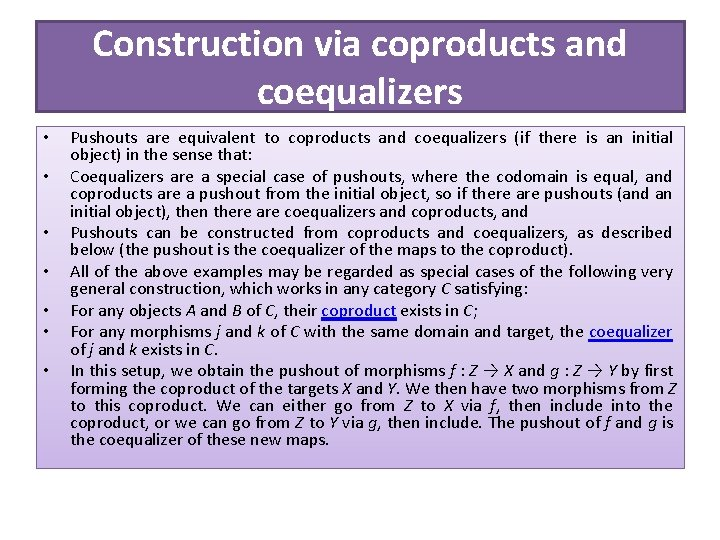Construction via coproducts and coequalizers • • Pushouts are equivalent to coproducts and coequalizers (if there is an initial object) in the sense that: Coequalizers are a special case of pushouts, where the codomain is equal, and coproducts are a pushout from the initial object, so if there are pushouts (and an initial object), then there are coequalizers and coproducts, and Pushouts can be constructed from coproducts and coequalizers, as described below (the pushout is the coequalizer of the maps to the coproduct). All of the above examples may be regarded as special cases of the following very general construction, which works in any category C satisfying: For any objects A and B of C, their coproduct exists in C; For any morphisms j and k of C with the same domain and target, the coequalizer of j and k exists in C. In this setup, we obtain the pushout of morphisms f : Z → X and g : Z → Y by first forming the coproduct of the targets X and Y. We then have two morphisms from Z to this coproduct. We can either go from Z to X via f, then include into the coproduct, or we can go from Z to Y via g, then include. The pushout of f and g is the coequalizer of these new maps.Monomorphism and Epimorphism • This page is about the mathematical term. For other uses, see Dimorphism (disambiguation) or Polymorphism (disambiguation). • In the context of abstract algebra or universal algebra, a monomorphism is an injective homomorphism. A monomorphism from X to Y is often denoted with the notation . • In the more general setting of category theory, a monomorphism (also called a monic morphism or a mono) is a left-cancellative morphism, that is, a map f : X → Y such that, for all morphisms g 1, g 2 : Z → X, • Monomorphisms are a categorical generalization of injective functions; in some categories the notions coincide, but monomorphisms are more general. • The categorical dual of a monomorphism is an epimorphism, i. e. a monomorphism in a category C is an epimorphism in the dual category Cop. Every section is a monomorphism, and every retraction is an epimorphism.Normal morphism • In category theory and its applications to mathematics, a normal monomorphism or normal epimorphism is a particularly wellbehaved type of morphism. A normal category is a category in which every monomorphism is normal. • Definition • A category C must have zero morphisms for the concept of normality to make complete sense. In that case, we say that a monomorphism is normal if it is the kernel of some morphism, and an epimorphism is normal (or conormal) if it is the cokernel of some morphism. • C itself is normal if every monomorphism is normal. C is conormal if every epimorphism is normal. Finally, C is binormal if it's both normal and conormal. But note that some authors will use only the word "normal" to indicate that C is actually binormal.Examples of normal morphisms • In the category of groups, a monomorphism f from H to G is normal if and only if its image is a normal subgroup of G. In particular, if H is a subgroup of G, then the inclusion map i from H to G is a monomorphism, and will be normal if and only if H is a normal subgroup of G. In fact, this is the origin of the term "normal" for monomorphisms. • On the other hand, every epimorphism in the category of groups is normal (since it is the cokernel of its own kernel), so this category is conormal. • In an abelian category, every monomorphism is the kernel of its cokernel, and every epimorphism is the cokernel of its kernel. Thus, abelian categories are always binormal. The category of abelian groups is the fundamental example of an abelian category, and accordingly every subgroup of an abelian group is a normal subgroup.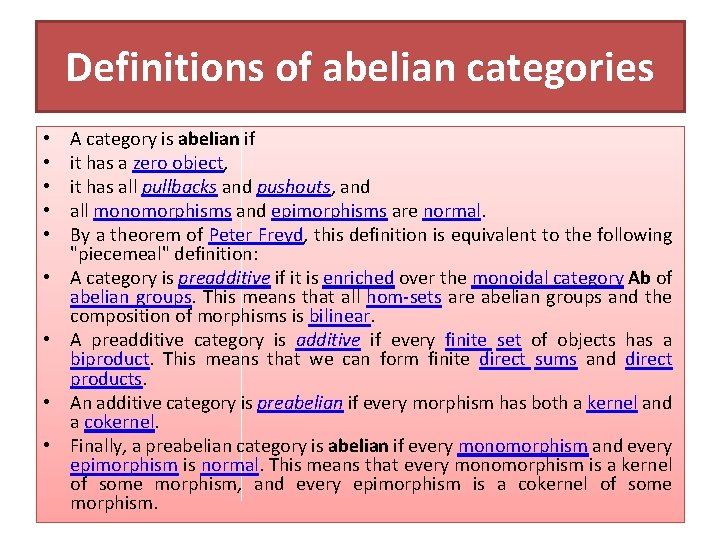Definitions of abelian categories • • • A category is abelian if it has a zero object, it has all pullbacks and pushouts, and all monomorphisms and epimorphisms are normal. By a theorem of Peter Freyd, this definition is equivalent to the following "piecemeal" definition: A category is preadditive if it is enriched over the monoidal category Ab of abelian groups. This means that all hom-sets are abelian groups and the composition of morphisms is bilinear. A preadditive category is additive if every finite set of objects has a biproduct. This means that we can form finite direct sums and direct products. An additive category is preabelian if every morphism has both a kernel and a cokernel. Finally, a preabelian category is abelian if every monomorphism and every epimorphism is normal. This means that every monomorphism is a kernel of some morphism, and every epimorphism is a cokernel of some morphism.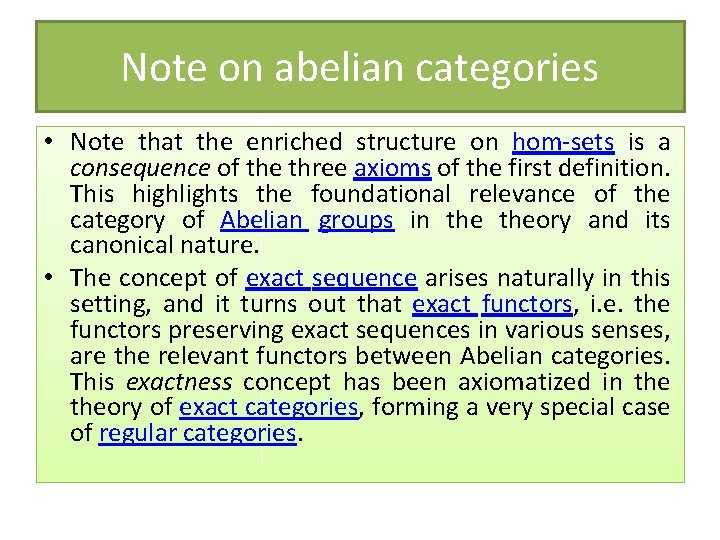Note on abelian categories • Note that the enriched structure on hom-sets is a consequence of the three axioms of the first definition. This highlights the foundational relevance of the category of Abelian groups in theory and its canonical nature. • The concept of exact sequence arises naturally in this setting, and it turns out that exact functors, i. e. the functors preserving exact sequences in various senses, are the relevant functors between Abelian categories. This exactness concept has been axiomatized in theory of exact categories, forming a very special case of regular categories.Examples of abelian categories • As mentioned above, the category of all abelian groups is an abelian category. The category of all finitely generated abelian groups is also an abelian category, as is the category of all finite abelian groups. • If R is a ring, then the category of all left (or right) modules over R is an abelian category. In fact, it can be shown that any small abelian category is equivalent to a full subcategory of such a category of modules (Mitchell's embedding theorem). • If R is a left-noetherian ring, then the category of finitely generated left modules over R is abelian. In particular, the category of finitely generated modules over a noetherian commutative ring is abelian; in this way, abelian categories show up in commutative algebra.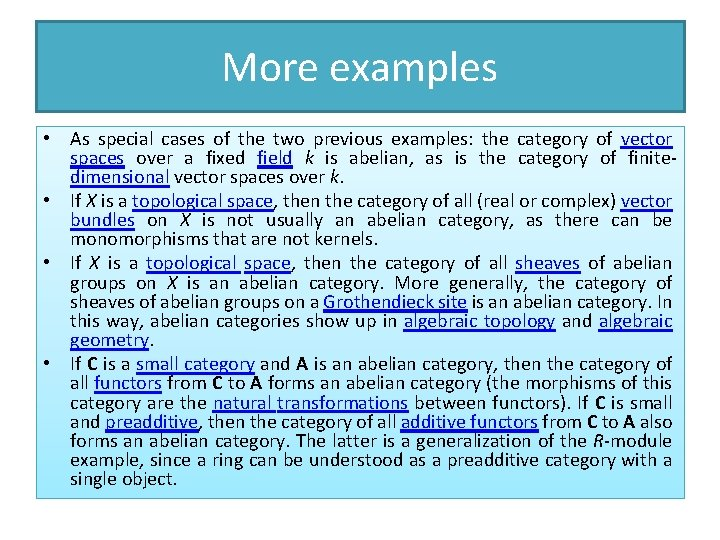More examples • As special cases of the two previous examples: the category of vector spaces over a fixed field k is abelian, as is the category of finitedimensional vector spaces over k. • If X is a topological space, then the category of all (real or complex) vector bundles on X is not usually an abelian category, as there can be monomorphisms that are not kernels. • If X is a topological space, then the category of all sheaves of abelian groups on X is an abelian category. More generally, the category of sheaves of abelian groups on a Grothendieck site is an abelian category. In this way, abelian categories show up in algebraic topology and algebraic geometry. • If C is a small category and A is an abelian category, then the category of all functors from C to A forms an abelian category (the morphisms of this category are the natural transformations between functors). If C is small and preadditive, then the category of all additive functors from C to A also forms an abelian category. The latter is a generalization of the R-module example, since a ring can be understood as a preadditive category with a single object.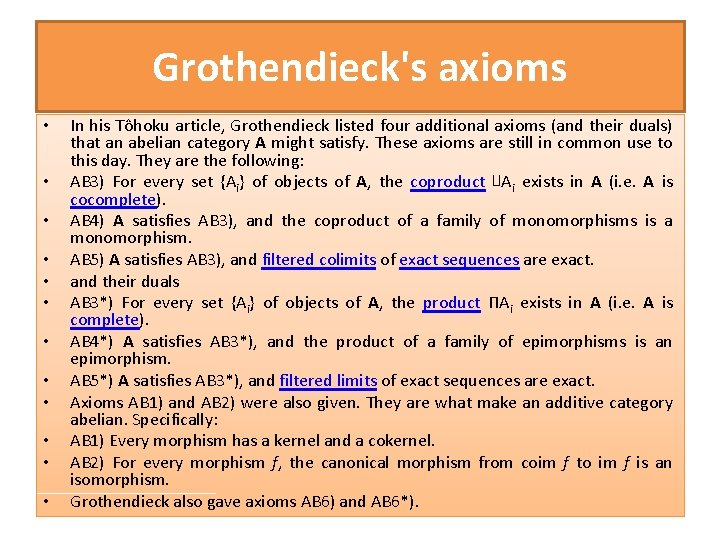Grothendieck's axioms • • • In his Tôhoku article, Grothendieck listed four additional axioms (and their duals) that an abelian category A might satisfy. These axioms are still in common use to this day. They are the following: AB 3) For every set {Ai} of objects of A, the coproduct ∐Ai exists in A (i. e. A is cocomplete). AB 4) A satisfies AB 3), and the coproduct of a family of monomorphisms is a monomorphism. AB 5) A satisfies AB 3), and filtered colimits of exact sequences are exact. and their duals AB 3*) For every set {Ai} of objects of A, the product ΠAi exists in A (i. e. A is complete). AB 4*) A satisfies AB 3*), and the product of a family of epimorphisms is an epimorphism. AB 5*) A satisfies AB 3*), and filtered limits of exact sequences are exact. Axioms AB 1) and AB 2) were also given. They are what make an additive category abelian. Specifically: AB 1) Every morphism has a kernel and a cokernel. AB 2) For every morphism f, the canonical morphism from coim f to im f is an isomorphism. Grothendieck also gave axioms AB 6) and AB 6*).Elementary properties of abelian categories • Given any pair A, B of objects in an abelian category, there is a special zero morphism from A to B. This can be defined as the zero element of the hom -set Hom(A, B), since this is an abelian group. Alternatively, it can be defined as the unique composition A → 0 → B, where 0 is the zero object of the abelian category. • In an abelian category, every morphism f can be written as the composition of an epimorphism followed by a monomorphism. This epimorphism is called the coimage of f, while the monomorphism is called the image of f. • Subobjects and quotient objects are well-behaved in abelian categories. For example, the poset of subobjects of any given object A is a bounded lattice. • Every abelian category A is a module over the monoidal category of finitely generated abelian groups; that is, we can form a tensor product of a finitely generated abelian group G and any object A of A. The abelian category is also a comodule; Hom(G, A) can be interpreted as an object of A. If A is complete, then we can remove the requirement that G be finitely generated; most generally, we can form finitary enriched limits in A.History of abelian categories • Abelian categories were introduced by Alexander Grothendieck in his famous Tôhoku paper in the middle of the 1950 s in order to unify various cohomology theories. At the time, there was a cohomology theory for sheaves, and a cohomology theory for groups. The two were defined completely differently, but they had formally almost identical properties. In fact, much of category theory was developed as a language to study these similarities. Grothendieck managed to unify the two theories: they both arise as derived functors on abelian categories; on the one hand the abelian category of sheaves of abelian groups on a topological space, on the other hand the abelian category of G-modules for a given group G.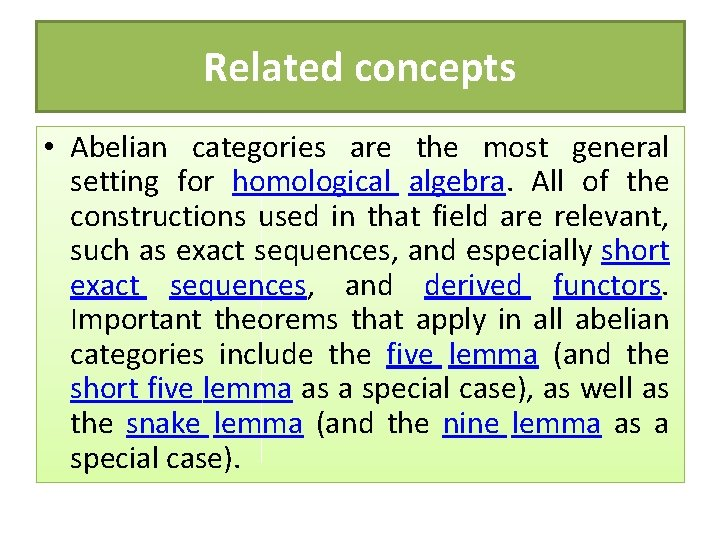Related concepts • Abelian categories are the most general setting for homological algebra. All of the constructions used in that field are relevant, such as exact sequences, and especially short exact sequences, and derived functors. Important theorems that apply in all abelian categories include the five lemma (and the short five lemma as a special case), as well as the snake lemma (and the nine lemma as a special case).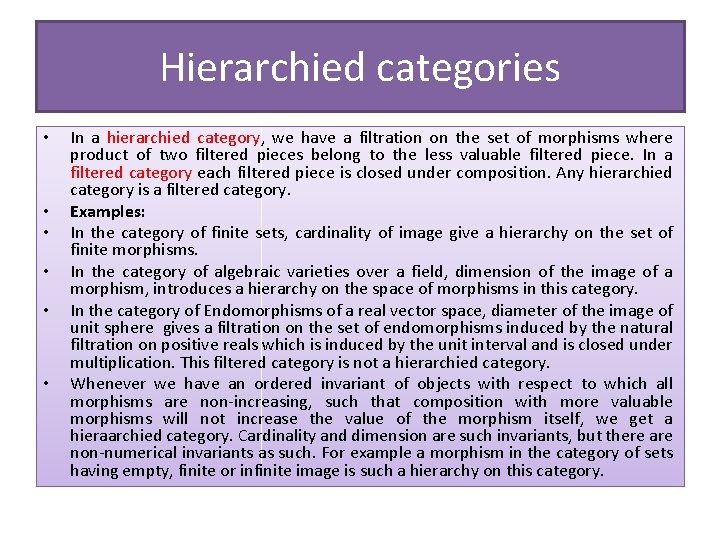Hierarchied categories • • • In a hierarchied category, we have a filtration on the set of morphisms where product of two filtered pieces belong to the less valuable filtered piece. In a filtered category each filtered piece is closed under composition. Any hierarchied category is a filtered category. Examples: In the category of finite sets, cardinality of image give a hierarchy on the set of finite morphisms. In the category of algebraic varieties over a field, dimension of the image of a morphism, introduces a hierarchy on the space of morphisms in this category. In the category of Endomorphisms of a real vector space, diameter of the image of unit sphere gives a filtration on the set of endomorphisms induced by the natural filtration on positive reals which is induced by the unit interval and is closed under multiplication. This filtered category is not a hierarchied category. Whenever we have an ordered invariant of objects with respect to which all morphisms are non-increasing, such that composition with more valuable morphisms will not increase the value of the morphism itself, we get a hieraarchied category. Cardinality and dimension are such invariants, but there are non-numerical invariants as such. For example a morphism in the category of sets having empty, finite or infinite image is such a hierarchy on this category.Hierarchied abelian categories • In a hierarchied abelian categories, having the model of finite dimensional vector spaces in mind, one expects more from a hierarchy: • Pullback of two morphisms should belong to the richer filtered piece of the two morphisms. So each filtered piece is closed under pullbacks. • Pushout of two morphisms should belong to the poorer filtered piece of the two morphisms. So each filtered piece is closed under pushouts. • Epimorphisms and monomorphisms induce a hierarchy on objects. Which is the same. • Example: • Rank of a morphism induces a hierarchy on the hierarchied abeliean category of finite dimensional vector spaces.Monoidal category • • • In mathematics, a monoidal category (or tensor category) is a category C equipped with a bifunctor ⊗ : C × C → C which is associative (up to a natural isomorphism), and an object I which is both a left and right identity for ⊗, (again, up to natural isomorphism). The associated natural isomorphisms are subject to certain coherence conditions which ensure that all the relevant diagrams commute. Monoidal categories are, therefore, a loose categorical analog of monoids in abstract algebra. The ordinary tensor product between vector spaces, abelian groups, R-modules, or R-algebras serves to turn the associated categories into monoidal categories. Monoidal categories can be seen as a generalization of these and other examples. In category theory, monoidal categories can be used to define the concept of a monoid object and an associated action on the objects of the category. They are also used in the definition of an enriched category. Monoidal categories have numerous applications outside of category theory proper. They are used to define models for the multiplicative fragment of intuitionistic linear logic. They also form the mathematical foundation for the topological order in condensed matter. Braided monoidal categories have applications in quantum field theory and string theory.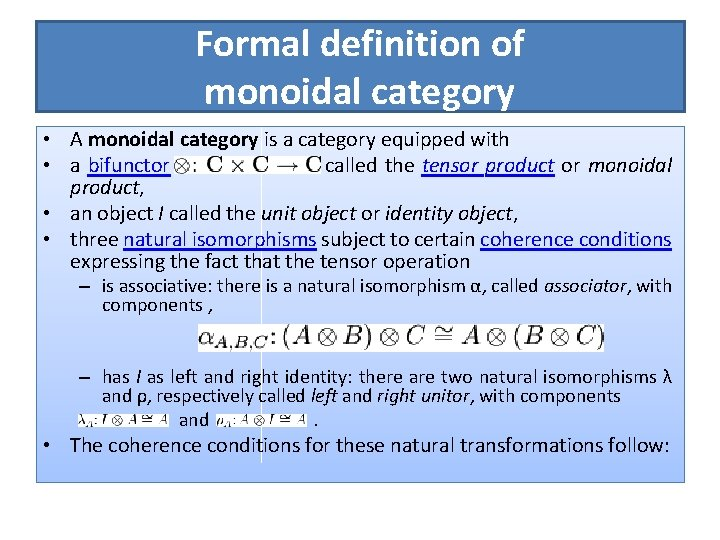Formal definition of monoidal category • A monoidal category is a category equipped with • a bifunctor called the tensor product or monoidal product, • an object I called the unit object or identity object, • three natural isomorphisms subject to certain coherence conditions expressing the fact that the tensor operation – is associative: there is a natural isomorphism α, called associator, with components , – has I as left and right identity: there are two natural isomorphisms λ and ρ, respectively called left and right unitor, with components and . • The coherence conditions for these natural transformations follow: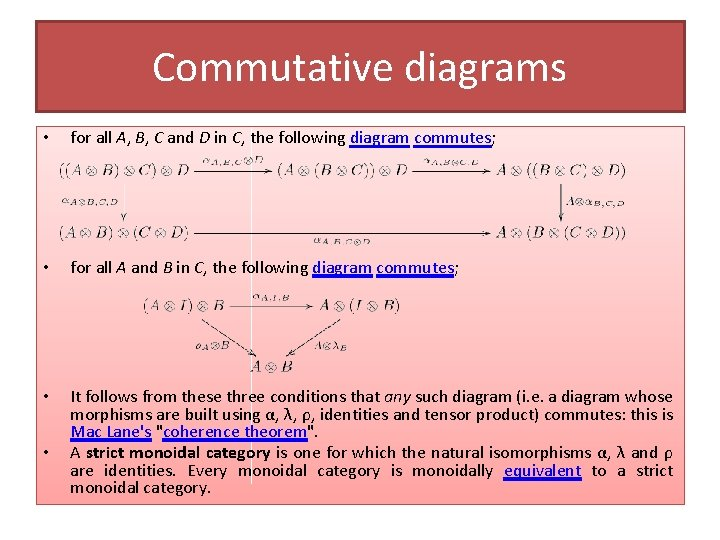Commutative diagrams • for all A, B, C and D in C, the following diagram commutes; • for all A and B in C, the following diagram commutes; • It follows from these three conditions that any such diagram (i. e. a diagram whose morphisms are built using α, λ, ρ, identities and tensor product) commutes: this is Mac Lane's "coherence theorem". A strict monoidal category is one for which the natural isomorphisms α, λ and ρ are identities. Every monoidal category is monoidally equivalent to a strict monoidal category. •Examples of monoidal categories • • • Any category with finite products is monoidal with the product as the monoidal product and the terminal object as the unit. Such a category is sometimes called a cartesian monoidal category. Any category with finite coproducts is monoidal with the coproduct as the monoidal product and the initial object as the unit. R-Mod, the category of modules over a commutative ring R, is a monoidal category with the tensor product of modules ⊗R serving as the monoidal product and the ring R (thought of as a module over itself) serving as the unit. As special cases one has: – K-Vect, the category of vector spaces over a field K, with the one-dimensional vector space K serving as the unit. – Ab, the category of abelian groups, with the group of integers Z serving as the unit. • • For any commutative ring R, the category of R-algebras is monoidal with the tensor product of algebras as the product and R as the unit. The category of pointed spaces is monoidal with the smash product serving as the product and the pointed 0 -sphere (a two-point discrete space) serving as the unit. The category of all endofunctors on a category C is a strict monoidal category with the composition of functors as the product and the identity functor as the unit. Bounded-above meet semilattices are strict symmetric monoidal categories: the product is meet and the identity is the top element.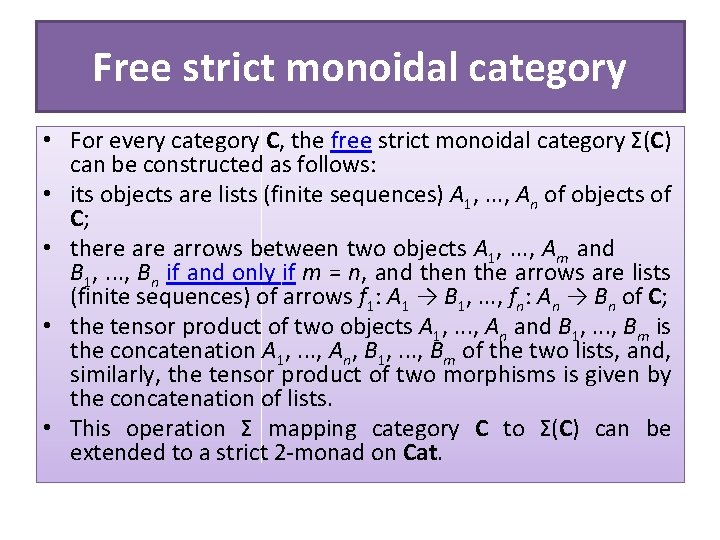Free strict monoidal category • For every category C, the free strict monoidal category Σ(C) can be constructed as follows: • its objects are lists (finite sequences) A 1, . . . , An of objects of C; • there arrows between two objects A 1, . . . , Am and B 1, . . . , Bn if and only if m = n, and then the arrows are lists (finite sequences) of arrows f 1: A 1 → B 1, . . . , fn: An → Bn of C; • the tensor product of two objects A 1, . . . , An and B 1, . . . , Bm is the concatenation A 1, . . . , An, B 1, . . . , Bm of the two lists, and, similarly, the tensor product of two morphisms is given by the concatenation of lists. • This operation Σ mapping category C to Σ(C) can be extended to a strict 2 -monad on Cat.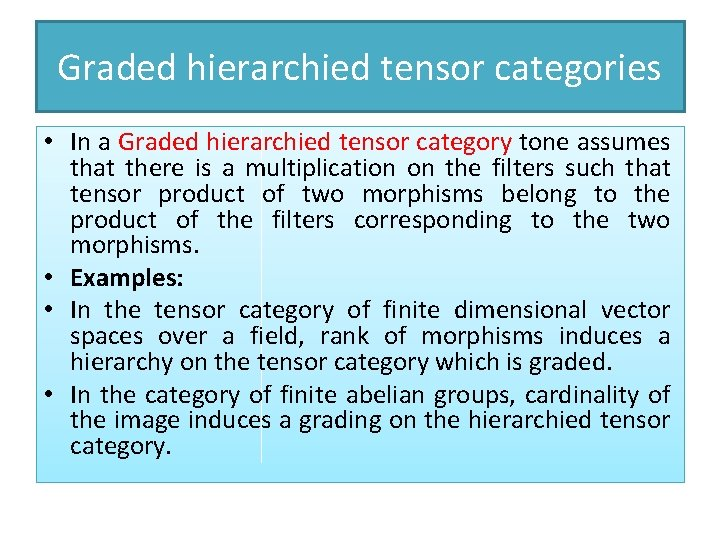Graded hierarchied tensor categories • In a Graded hierarchied tensor category tone assumes that there is a multiplication on the filters such that tensor product of two morphisms belong to the product of the filters corresponding to the two morphisms. • Examples: • In the tensor category of finite dimensional vector spaces over a field, rank of morphisms induces a hierarchy on the tensor category which is graded. • In the category of finite abelian groups, cardinality of the image induces a grading on the hierarchied tensor category.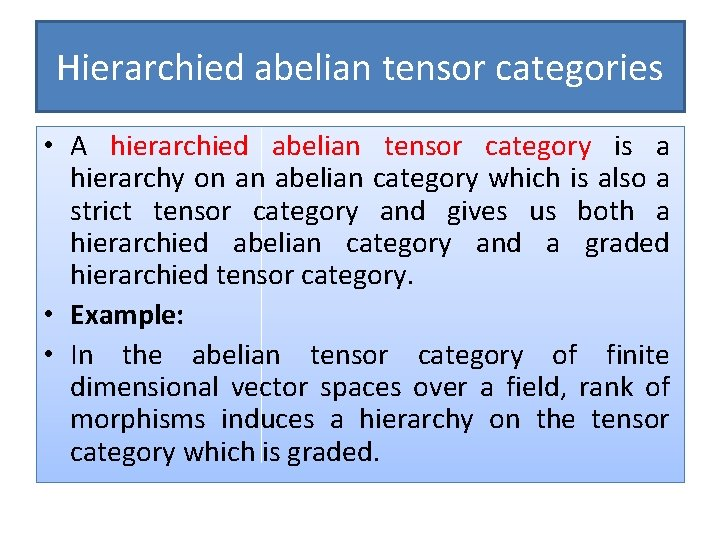Hierarchied abelian tensor categories • A hierarchied abelian tensor category is a hierarchy on an abelian category which is also a strict tensor category and gives us both a hierarchied abelian category and a graded hierarchied tensor category. • Example: • In the abelian tensor category of finite dimensional vector spaces over a field, rank of morphisms induces a hierarchy on the tensor category which is graded.